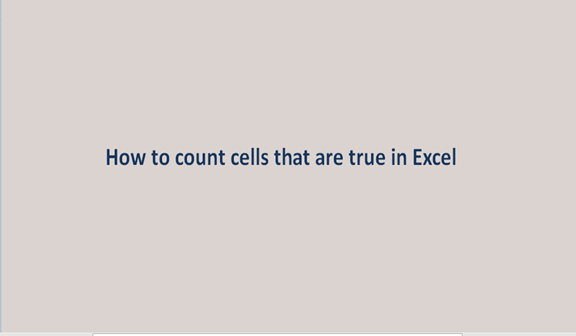## How to count cells that are true in Excel

The act of tallying a certain quantity to obtain or to reveal the number of items or several objects in a set is what we mostly refer to as counting. Counting is general and done in almost all aspects of life or our daily routines.

In most cases, we count in numbers that are to mean we use numbers to identify the number of items or the number of objects. In excel sheets we do even the count of the number of true entries in cells.

To count cells that are true means that we find the tally of cells that their data or inputs when viewed or looked into giving a true result. An example can be the name Mary is of the female gender. This statement is true under all circumstances and all conditions.

Since we are interested in the count of all true values in a given data set, the value that appears in the result cell will only be a digit or a number.

To find the number of true cells in any given excel sheet, we have to follow a series of procedures which may include the following

Step 1

Insert data in an empty excel sheet with three columns, one for names, the other for gender, and the other one for decision. To do this, on your laptop or tablet, search for Microsoft Excel and open it, a blank sheet will open where you will enter your data. A sample is shown below in the screenshot.Step 2

To get the count of all the true entries in the scenario above, we will use the function COUNTIF. On the formula bar or the result cell type in the formula =COUNTIF (A2: C5, TRUE) and click on the enter button.

To count the tally of all the TRUE entries which in the scenario is 4, we will use the above formula. The screenshot below gives you the full idea and explanation in full detail.We can as well as count physically but because excel gives us the capability to calculate or the automatic calculations, we ought to use the formulas to make our work easy and avoid human-related errors.